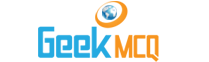# CSS :: Equilibrium of firm Perfect Competition and Monopoly

1.  A firm decides to exit the industry when:
 A. AC strts rising B. MC starts rising C. Price is less than LAC D. TC starts rising

2.  Profit is maximum when:
 A. TC and TR curves are parallel B. MC and MR curves are parallel C. TC and TR curves cross each other D. AC and AR curves cross each other

3.  In monopoly and perfect competition the cost curves are:
 A. Same B. Different C. Opposite D. None of these

4.  Normal profit is called normal because:
 A. It is neither very high nor very low B. It is minimum acceptable to the producer C. It is minimum which buyer wants to pay D. It is the maximum allowed by govt.

5.  If a firm shuts down temporarily, it will incur loss equal to:
 A. AFC B. AVC C. TFC D. TVC

6.  Under perfect competition:
 A. AC = AVC B. AR = AC C. AR = MC D. AR = MR

7.  The necessary condition for equilibrium position of a firm is:
 A. MR = MC B. MC > Price C. MC = MR D. MC = AC

8.  When a competitive firm achieves long run equilibrium then:
 A. P = MC B. MR = MC C. P = ATC D. All of the above

9.  The most efficient scale of production of a firm is where:
 A. LAC is minimum B. SAC is minimum C. LMC is minimum D. SMC is minimum

10.  A firm should shut down in the short run if it is not covering its:
 A. Variable cost B. Fixed cost C. Total cost D. Explicit cost (money outlays)